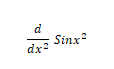How to find derivative of a function in python

In this section, We discuss the Differentiation of equation. The main goal of this section is a way to find a derivative of a function in Python. But before moving to the coding part first you should aware of the derivatives of a function. In this, we used sympy library to find a derivative of a function in Python.

Derivatives described as how you calculate the rate of a function at a given point. For example, acceleration is the derivative of speed.

If you have got a function which will be expressed as f (x) = 2x^2 + 3, then the derivative of that function, or the rate at which that function is changing, is calculated as f '(x) = Can be done with 4x.

Many programming languages include libraries to do more complex mathematics.

One topic many programming languages have difficulty with is symbolic mathematics. If you use Python though, you have access to the symbolic mathematics library Sympy. It is written entirely in Python, so you will not need to install any additional requirements.

If you want the latest and greatest, you can download a source tarball or git repository. Once it is installed, you will be able to access the Sympy library in two ways. You can access it like any other library with import details.

Sympy also provides a binary known as isympy which is modeled after the ipython.

Sympy can be used as a calculator. Sympy has built-in support for three numeric types are given below:

float, rational, and integer.

Float and integer are comfortable, but what is rational? A rational number is formed from a numerator and a denominator.

So, rational (5,2) is equal to 5/2. There is also support for complex numbers. The imaginary part of a complex number is tagged with a constant I.

If you want to know how to install and import sympy in Python then you must check Python libraries. Here you can check how to install and import Sympy library in Python in an easy way.

## Derivatives

To take derivatives, use the diff function.

Let's take a look at how to Differentiation can find out using Sympy. Differentiation can be expressed in three ways:
1. diff(f)
2. diff(f,var)
3. diff(f,x,1)

1. Differentiation for sin(x)
```from sympy import *
x = symbols('x')
f = sin(x)
y = diff(f)
print(y)
Output: cos(x)
from sympy import *
x = symbols('x')
f = sin(x)
y = diff(f,x)
print(y)
Output: cos(x)
from sympy import *
x = symbols('x')
f = sin(x)
y = diff(f,x,1)
print(y)
Output: cos(x)

from sympy import *
x = symbols('x')
f = sin(x)
y = diff(f,x,2)
print(y)
Output: cos(x)

2. Differentiation
for sin(2x)
from sympy import *
x = symbols('x')
f = sin(2*x)
y = diff(f,x)
print(y)
Output: 2*cos(2*x)

3. Differentiation for tan(x)

from sympy import *
x = symbols('x')
f = tan(x)
y = diff(f,x)
print(y)

Output:tan(x)**2 + 1

4. Differentiation for the given equation using sympy.from sympy import *
x = symbols('x')
f = (exp(x)*cos(x))
y = diff(f,x)
print(y)
Output: -exp(x)*sin(x) + exp(x)*cos(x)

5. Differentiation for the given equation using sympy.from sympy import *
x = symbols('x')
f = sin(x**2)
y = diff(f,x)
print(y)

Output: 2*x*cos(x**2)

6. Differentiation for the given equation using sympy.from sympy import *
x = symbols('x')
h = symbols('h')
f = sin(x*h**2)
y = diff(f,x,1)
print(y)
Output: h**2*cos(h**2*x)

7. Differentiation for the given equation using sympy.from sympy import *
x = symbols('x')
h = symbols('h')
f = sin(x*h**2)
y = diff(f,x,2)
print(y)
Output: -h**4*sin(h**2*x)

8. Differentiation for the given equation using sympy.from sympy import *
x = symbols('x')
h = symbols('h')
f = sin(x**2)
y = diff(f,x)
print(y)
Output: 2*x*cos(x**2)

9. Differentiation for the given equation using sympy.from sympy import *
x = symbols('x')
f = ((4*(x**3)-(2*x)-6)/(-(x**3)+(x**2)-1))
y = diff(f,x)
print(y)
Output: (3*x**2 - 2*x)*(4*x**3 - 2*x - 6)/(-x**3 + x**2 - 1)**2 + (12*x**2 - 2)/(-x**3 + x**2 - 1)

```